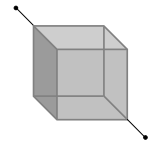Twelve wires of equal length and same cross-section are connected in the form of a cube. If the resistance of each of the wires is R, then the effective resistance between the two diagonal ends would be(1) 2R

(2) 12R

(3) $\frac{5}{6}R$

(4) 8R